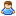1.You are currently browsing our forum as a guest. Create your own forum account to access all forum functionality.

# How to get current input of power consumer block?

Discussion in 'Programming Questions and Suggestions' started by meloncrashed, Dec 6, 2017.

This last post in this thread was made more than 31 days old.
1. ### meloncrashedTrainee Engineer

Messages:
3
I can get Max Required Input, Current Output and Max Out from block.DetailedInfo.
Is there any property or method to get current input from power consumer block?

2. Partial code Snipped from: https://github.com/Wicorel/WicoSpaceEngineers/blob/master/Action displayer V2.2.cs#L414

Code:
```	Echo("Accepted resources:");
values.Append("\nAccepted resources:");
MyResourceSinkComponent sink;
unit.Components.TryGet<MyResourceSinkComponent>(out sink);
if (sink != null)
{
var list = sink.AcceptedResources;
for (int j = 0; j < list.Count; ++j)
{
values.Append("\n " + list[j].SubtypeId.ToString() +" ("+list[j].SubtypeName+")");
Echo(list[j].SubtypeId.ToString() +" ("+list[j].SubtypeName+")");

float currentInput = 0;
float maxRequiredInput = 0;
bool isPoweredBy = false;

currentInput=sink.CurrentInputByType(list[j]);
isPoweredBy=sink.IsPoweredByType(list[j]);
maxRequiredInput=sink.MaxRequiredInputByType(list[j]);

values.Append("\n Current=" + currentInput.ToString() + " Max=" + maxRequiredInput.ToString() + " Is=" + isPoweredBy.ToString());

}
}
else
{
values.Append("\n No Resources");
Echo("No resources");
}

```